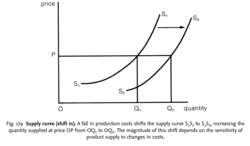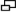# supply curve

Also found in: Dictionary, Encyclopedia.
Related to supply curve: Supply Schedule

## supply curve

see EQUILIBRIUM MARKET PRICE.

## supply curve

a line showing the relationship between the PRICE of a PRODUCT or FACTOR OF PRODUCTION and the quantity supplied per time period. ‘Supply’ means the total quantity of a product or factor that firms or factor owners are prepared to sell at a given price.

The typical market supply curve for a product slopes upwards from left to right, indicating that as price rises more is supplied (that is, a movement along the existing supply curve). Thus, if price rises from OP1, to OP2 in Fig. 178, the quantity supplied will increase from OQ1, to OQ2 .

Fig. 178 shows the supply curve for the market as a whole. This curve is derived by aggregating the individual supply curves of all the producers of the good, which in turn are derived from the producers’ cost curves.

Most supply curves slope upwards because, as the price of the product rises, producers will find it more profitable to manufacture, using their existing production facilities, and because any increase in short-run MARGINAL COSTS associated with increasing output will be covered by the higher price obtained.

The slope of the supply curve reflects the degree of responsiveness of quantity supplied to changes in the product's price. For example, if a large rise in price results in only a small increase in quantity supplied (as would be the case where the supply curve has a steep slope), then supply is said to be price-inelastic (see PRICE-ELASTICITY OF SUPPLY).

The supply curve interacts with the DEMAND CURVE to determine the EQUILIBRIUM MARKET PRICE. See SUPPLY CURVE, (SHIFT IN), SUPPLY FUNCTION.Fig. 179 Supply curve (shift in). A fall in production costs shifts the supply curve S1 S1 to S2 S2, increasing the quantity supplied at price OP from OQ1 to OQ2. The magnitude of this shift depends on the sensitivity of product supply to changes in costs.

## supply curve (shift in)

a movement of the SUPPLY CURVE from one position to another (either left or right) as a result of some economic change, other than PRICE. A given supply curve is always drawn on the CETERIS PARIBUS assumption that all the other factors affecting supply (costs in particular) are held constant. If any of these change, however, then this will bring about a shift in the supply curve. For example, if costs of production fall, the supply curve will shift to the right (see Fig. 179) so that more is now supplied at each price than formerly. See also SUPPLY FUNCTION.
References in periodicals archive ?
A second is that this explanation implies that there is a discontinuity between willingness to pay and willingness to accept, with a vertical gap in the labor- supply curve.
The novel twist today is the ever-expanding global supply curve.
2) When the slope absolute value of supply curve is less than demand curve, it means supply elasticity is large.
33 per mcf) and the 2007 supply curve (which we take to be the counterfactual 2013 supply curve), calculate the area bounded by the 2013 price (\$3.
As part of the video, Solman and his guests debate the shape of the Oprah Supply Curve.
A change in quantity supplied is a move along a given supply curve in response to different prices.
We measure oligopsony market power, computing the hourly price elasticity of the ex-post residual supply curve for each consumer, which is the difference between total supply and demand of all other consumers.
Graphically, the change in time preference is generally visualized as a movement in the supply curve along a constant demand curve in the loanable funds market, which implies a similar shift in the overall time market (Skousen, 2007, p.
The supply curve can be derived by calculating the value of [[theta].
Some executives also consider capacity that is "out of the market" and evaluate how a reduction in volume might enable higher pricing by "pulling" the supply curve to the left.
While in most markets an upward sloping supply curve would be unsurprising, in the health service market, this means that physicians treat patients according to service price levels.

Site: Follow: Share:
Open / Close